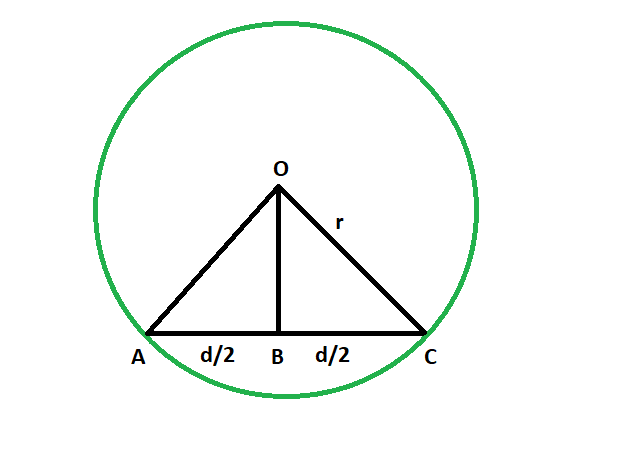# Shortest distance from the center of a circle to a chord

Given a circle which has a chord inside it. The length of the chord and the radius of the circle are given. The task is to find the shortest distance from the chord to the center.
Examples:

```Input: r = 4, d = 3
Output: 3.7081

Input: r = 9.8, d = 7.3
Output: 9.09492```Approach

• We know that the line segment that is dropped from the center on the chord bisects the chord. The line is the perpendicular bisector of the chord, and we know the perpendicular distance is the shortest distance, so our task is to find the length of this perpendicular bisector.
• let radius of the circle = r
• length of the chord = d
• so, in triangle OBC,
from Pythagoras theorem
OB^2 + (d/2)^2 = r^2
so, OB = √(r^2 – d^2/4)
• So,Below is the implementation of the above approach:

## C++

 `// C++ program to find` `// the shortest distance from` `// chord to the center of circle` `#include ` `using` `namespace` `std;`   `// Function to find the shortest distance` `void` `shortdis(``double` `r, ``double` `d)` `{` `    ``cout << ``"The shortest distance "` `         ``<< ``"from the chord to center "` `         ``<< ``sqrt``((r * r) - ((d * d) / 4))` `         ``<< endl;` `}`   `// Driver code` `int` `main()` `{` `    ``double` `r = 4, d = 3;` `    ``shortdis(r, d);` `    ``return` `0;` `}`

## Java

 `// Java program to find ` `// the shortest distance from ` `// chord to the center of circle ` `class` `GFG` `{` `    `  `// Function to find the shortest distance ` `static` `void` `shortdis(``double` `r, ``double` `d) ` `{ ` `    ``System.out.println(``"The shortest distance "` `        ``+ ``"from the chord to center "` `        ``+ (Math.sqrt((r * r) - ((d * d) / ``4``)))); ` `} `   `// Driver code ` `public` `static` `void` `main(String[] args)` `{` `    ``double` `r = ``4``, d = ``3``; ` `    ``shortdis(r, d); ` `}` `}`   `/* This code contributed by PrinciRaj1992 */`

## Python3

 `# Python program to find ` `# the shortest distance from ` `# chord to the center of circle `   `# Function to find the shortest distance ` `def` `shortdis(r, d): ` `    ``print``(``"The shortest distance "``,end``=``"");` `    ``print``(``"from the chord to center "``,end``=``"");` `    ``print``(((r ``*` `r) ``-` `((d ``*` `d) ``/` `4``))``*``*``(``1``/``2``));`   `# Driver code ` `r ``=` `4``;` `d ``=` `3``; ` `shortdis(r, d); `   `# This code has been contributed by 29AjayKumar`

## C#

 `// C# program to find ` `// the shortest distance from ` `// chord to the center of circle ` `using` `System;`   `class` `GFG ` `{ ` `    `  `    ``// Function to find the shortest distance ` `    ``static` `void` `shortdis(``double` `r, ``double` `d) ` `    ``{ ` `        ``Console.WriteLine(``"The shortest distance "` `            ``+ ``"from the chord to center "` `            ``+ (Math.Sqrt((r * r) - ((d * d) / 4)))); ` `    ``} ` `    `  `    ``// Driver code ` `    ``public` `static` `void` `Main() ` `    ``{ ` `        ``double` `r = 4, d = 3; ` `        ``shortdis(r, d); ` `    ``} ` `} `   `// This code is contributed by AnkitRai01`

## PHP

 ``

## Javascript

 ``

Output

```The shortest distance from the chord to center 3.7081
```

Time Complexity: O(1)

Auxiliary Space: O(1)

Feeling lost in the world of random DSA topics, wasting time without progress? It's time for a change! Join our DSA course, where we'll guide you on an exciting journey to master DSA efficiently and on schedule.
Ready to dive in? Explore our Free Demo Content and join our DSA course, trusted by over 100,000 geeks!

Previous
Next